Open in App
Not now

# Julia Dictionary

• Difficulty Level : Basic
• Last Updated : 22 Apr, 2020

Dictionary in Julia is a collection of key-value pairs, where each value in the dictionary can be accessed with its key. These key-value pairs need not be of the same data type, which means a String typed key can hold a value of any type like Integer, String, float, etc. Keys of a dictionary can never be same, each key must be unique. This doesn’t apply to the values, values can be same, as per need. Dictionaries by default are an unordered collection of data i.e. it doesn’t maintain the order in which keys are inserted.

A dictionary is more like an array but in a dictionary, the indices can be of any type while in an array, the indices have to be integers only.
Each key in a dictionary maps to a value. Because the keys need to be unique, two keys can be mapped with two same values but two different values can’t be mapped with a single key.

Syntax:

Dictionary_name = Dict(“key1” => value1, “key2” => value2, …)

#### Creating a Dictionary

A dictionary in Julia can be created with a pre-defined keyword Dict(). This keyword accepts key-value pairs as arguments and generates a dictionary by defining its data type based on the data type of the key-value pairs. One can also pre-define the data type of the dictionary if the data type of the values is known. This can be done by defining the data type within curly braces after the `Dict` keyword. This dictionary with pre-defined data types is known as Typed Dictionary.

Syntax:

```Dictionary_name = Dict{Key_datatype, Value_datatype}("Key1" => value1, "Key2" => value2, ...)
```

Example:

 `# Julia program to illustrate ``# the use of Dictionary`` ` `# Creating an Empty dictionary``Dict1 ``=` `Dict``()``println(``"Empty Dictionary = "``, Dict1)`` ` `# Creating an Untyped Dictionary``Dict2 ``=` `Dict``(``"a"` `=``> ``1``, ``"b"` `=``> ``2``, ``"c"` `=``> ``3``)``println(``"\nUntyped Dictionary = "``, Dict2)`` ` `# Creating a Typed Dictionary``Dict3 ``=` `Dict``{String, Integer}(``"a"` `=``> ``10``, ``"c"` `=``> ``20``)``println(``"\nTyped Dictionary = "``, Dict3)`

Output: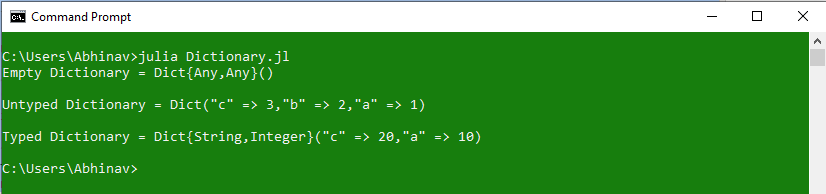#### Accessing elements from a Dictionary

Elements from a dictionary can be accessed by using the keys of the dictionary. These keys are unique in a dictionary and hence each key holds a single value. A key-value pair can also be accessed with the use of for-loop.

Syntax:

```Dictionary_name[key_name]
or
Dictionary_name[:key_name]
```

Example:

 `# Julia program to illustrate ``# the use of Dictionary`` ` `# Creating a Dictionary with String keys``Dict1 ``=` `Dict``(``"a"` `=``> ``1``, ``"b"` `=``> ``2``, ``"c"` `=``> ``"Hello"``)`` ` `# Accessing dictionary values using keys``println(Dict1[``"b"``])``println(Dict1[``"c"``])`` ` `# Creating a Dictionary with Integer keys``Dict2 ``=` `Dict``(``1` `=``> ``10``, ``2` `=``> ``20``, ``3` `=``> ``"Geeks"``)``println(Dict2[``1``])``println(Dict2[``3``])`` ` `# Creating a Dictionary with Symbols``Dict3 ``=` `Dict``(:a ``=``> ``1``, :b ``=``> ``"one"``)``println(Dict3[:b])`

Output: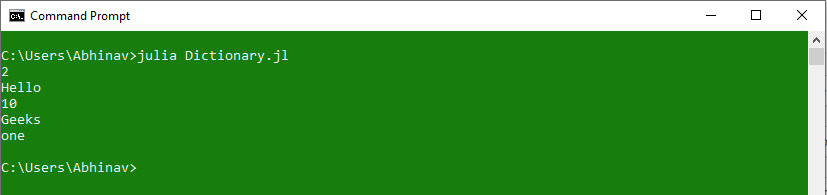Use of `get()` function:
Julia provides a pre-defined function to access elements of a Dictionary known as `get()` function. This function takes 3 arguments: dictionary name, key, and a default value to print if the key is not found.
Syntax:

```get(Dictionary_name, Key_name, Default Value)
```

Example:

 `# Julia program to illustrate ``# the use of Dictionary`` ` `# Creating a Dictionary with mixed-typed keys``Dict1 ``=` `Dict``(``"a"` `=``> ``1``, ``"b"` `=``> ``2``, ``"c"` `=``> ``"Hello"``, ``4` `=``> ``10``)`` ` `# Accessing using get() function`` ` `# Passing '0' as default value ``println(get(Dict1, ``"b"``, ``0``))`` ` `# Passing String as Default value``println(get(Dict1, ``"d"``, ``"Sorry, no such key"``))`

Output: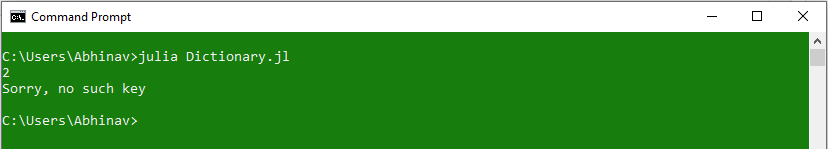#### Accessing Keys and Values from a Dictionary:

Dictionaries in Julia allow accessing all the keys and all the values at once. This can be done with the use of pre-defined keywords `keys` and `values`.

Syntax:

```Keys = keys(Dictionary_name)
Values = values(Dictionary_name)
```

Example:

 `# Julia program to illustrate ``# the use of Dictionary`` ` `# Creating a Dictionary with mixed-typed keys``Dict1 ``=` `Dict``(``"a"` `=``> ``1``, ``"b"` `=``> ``2``, ``"c"` `=``> ``"Hello"``, ``4` `=``> ``10``)`` ` `# Accessing all the Keys``# using 'keys' keyword``Keys ``=` `keys(Dict1)``println(``"Keys = "``, Keys)`` ` `# Accessing all the Values``# using 'values' keyword``Values ``=` `values(Dict1)``println(``"Values = "``, Values)`

Output: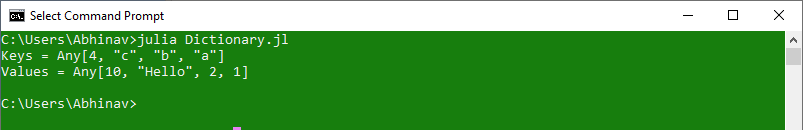Printing Key-value pairs:
All the Key-value pairs of a dictionary can be printed at once, with the use of for-loop. This is done by iterating over each key of the dictionary and then accessing the respective value of that key.

Example 1: Using Dictionary as iterable object

 `# Julia program to illustrate ``# the use of Dictionary`` ` `# Creating a Dictionary with mixed-typed keys``Dict1 ``=` `Dict``(``"a"` `=``> ``1``, ``"b"` `=``> ``2``, ``"c"` `=``> ``"Hello"``, ``4` `=``> ``10``)`` ` `# Printing key-value pair using``# Dictionary as an iterable object``for` `i ``in` `Dict1``    ``println(i)``end`

Output: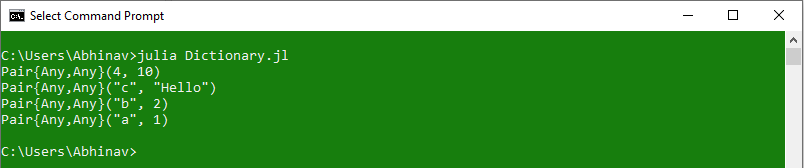Example 2: Accessing each key one by one

 `# Julia program to illustrate ``# the use of Dictionary`` ` `# Creating a Dictionary with mixed-typed keys``Dict1 ``=` `Dict``(``"a"` `=``> ``1``, ``"b"` `=``> ``2``, ``"c"` `=``> ``"Hello"``, ``4` `=``> ``10``)`` ` `# Printing key-value pair by``# accessing each key one-by-one``for` `i ``in` `keys(Dict1)``    ``println(i, ``" => "``, Dict1[i])``end`

Output: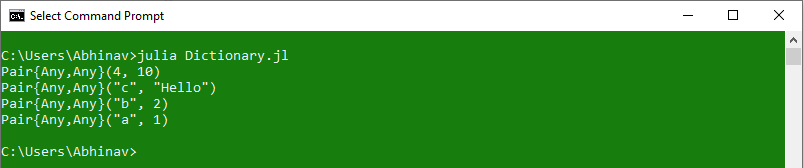Example 3: By using (key, value) tuples

 `# Julia program to illustrate ``# the use of Dictionary`` ` `# Creating a Dictionary with mixed-typed keys``Dict1 ``=` `Dict``(``"a"` `=``> ``1``, ``"b"` `=``> ``2``, ``"c"` `=``> ``"Hello"``, ``4` `=``> ``10``)`` ` `# Printing key-value pair by``# using key and value tuples``for` `(i, j) ``in` `Dict1``    ``println(i, ``" => "``, j)``end`

Output: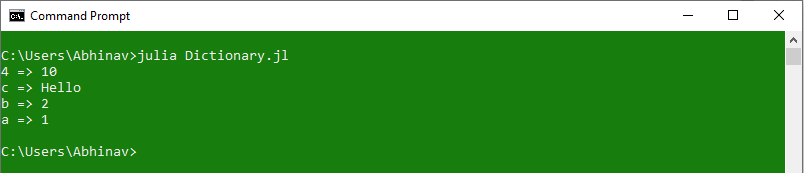#### Modifying elements of a Dictionary

Modification of elements of a Dictionary includes the process of adding new keys, modifying existing key values, and deleting a key. Modification of elements doesn’t include renaming keys of the dictionary, however, it can be done by deleting the existing key and adding another key with the same value.

 `# Julia program to illustrate ``# the use of Dictionary`` ` `# Creating a Dictionary with mixed-typed keys``Dict1 ``=` `Dict``(``"a"` `=``> ``1``, ``"b"` `=``> ``2``, ``"c"` `=``> ``"Hello"``, ``4` `=``> ``10``)``println(``"Initial Dictionary: \n"``, Dict1)`` ` `# Adding a new key``Dict1[``"d"``] ``=` `20``println(``"\nUpdated Dictionary after Adding new key: \n"``, Dict1)`` ` `# Updating existing key``Dict1[``"c"``] ``=` `"Hello Geeks"``println(``"\nUpdated Dictionary after Updating a key: \n"``, Dict1)`` ` `# Deleting an existing key``Dict1 ``=` `delete !(Dict1, ``"d"``)``println(``"\nUpdated Dictionary after Deleting a key: \n"``, Dict1)`

Output: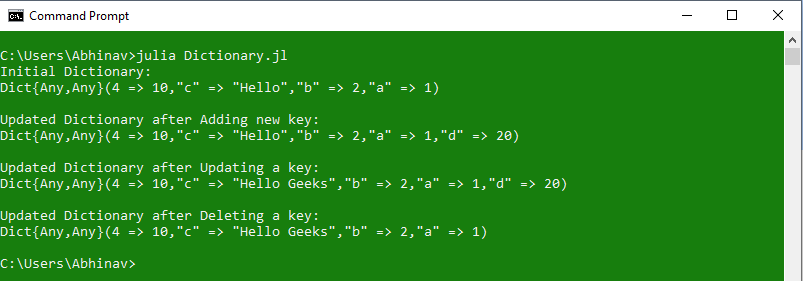#### Dictionary Methods

MethodsDescription
get()Used to return the value stored for the specified key, or the given default value if no mapping for the key is present.
get!()Used to return the value stored for the specified key, or if no mapping for the key is present, store key => default, and return default.
getkey()Used to return the key matching argument key if one exists in collection, otherwise return default.
keytype()Used to return the key type of the specified array.
merge()Used to construct a merged collection from the specified collections
merge!()Used to update collection with pairs from the other collections.
pairs()Returns an iterator over key=>value pairs for the specified collection that maps a set of keys to a set of values.
valtype()Used to return the value type of the specified array.

My Personal Notes arrow_drop_up